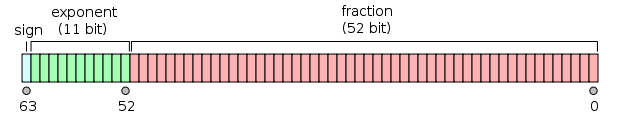# C++ 变量类型

C++ 变量类型

bool 存储值 true 或 false。
char 通常是一个字符（八位）。这是一个整数类型。
int 对机器而言，整数的最自然的大小。
float 单精度浮点值。单精度是这样的格式，1位符号，8位指数，23位小数。doublevoid 表示类型的缺失。
wchar_t 宽字符类型。

C++ 也允许定义各种其他类型的变量，比如枚举、指针、数组、引用、数据结构、类等等，这将会在后续的章节中进行讲解。

## C++ 中的变量定义

type variable_list;


int    i, j, k;
char   c, ch;
float  f, salary;
double d;


int i, j, k; 声明并定义了变量 i、j 和 k，这指示编译器创建类型为 int 的名为 i、j、k 的变量。

type variable_name = value;


extern int d = 3, f = 5;    // d 和 f 的声明
int d = 3, f = 5;           // 定义并初始化 d 和 f
byte z = 22;                // 定义并初始化 z
char x = 'x';               // 变量 x 的值为 'x'


## 实例

#include <iostream>
using namespace std;

// 变量声明
extern int a, b;
extern int c;
extern float f;

int main ()
{
// 变量定义
int a, b;
int c;
float f;

// 实际初始化
a = 10;
b = 20;
c = a + b;

cout << c << endl ;

f = 70.0/3.0;
cout << f << endl ;

return 0;
}


30
23.3333


// 函数声明
int func();

int main()
{
// 函数调用
int i = func();
}

// 函数定义
int func()
{
return 0;
}


## C++ 中的左值（Lvalues）和右值（Rvalues）

C++ 中有两种类型的表达式：

• 左值（lvalue）：指向内存位置的表达式被称为左值（lvalue）表达式。左值可以出现在赋值号的左边或右边。
• 右值（rvalue）：术语右值（rvalue）指的是存储在内存中某些地址的数值。右值是不能对其进行赋值的表达式，也就是说，右值可以出现在赋值号的右边，但不能出现在赋值号的左边。

int g = 20;


10 = 20;


• 回顶